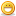# Thread: Value of a single variable (column) into data table

1. ## Value of a single variable (column) into data table

How do we write the value of a single variable (column) into the data table?Reply With Quote

2. ## Re: Value of a single variable (column) into data table

Well ur Q is not very clear but if you mean how you can pass some value from the test to the Data sheet then you can use the getParameter function
Ex:
DataTable.GetSheet("Global").GetParameter("Create_Product").Value="pass the value here"

Let me know if you have any Q or if i misunderstand your question.

Thanks,
Kunal ShekharReply With Quote

3. ## Re: Value of a single variable (column) into data table

Hi Kunal, I have used this code i replaced the column name with RequestNumber.
but though the test passed, it does not put the value to the data table.

The variable to which value is assigned is reqno. and column name is RequestNumber.
and reqno is the variable which has value REQ0043215,Now i need to display REQ0043215 in the datatable.
so i wrote the code as

DataTable.GetSheet("Global").GetParameter("RequestNumber").Value="Reqno"
Can you please identify where am i going wrong?Reply With Quote

4. ## Re: Value of a single variable (column) into data table

I don know what exactle you want to achieve but in this case the error is
DataTable.GetSheet("Global").GetParameter("RequestNumber").Value="Reqno"
remove the double quotes("") from reqno. and it will print the value in the datatable.

then if you want to keep the values alive after test is complete then you need to export the excel sheet. whihc is a simple command.
Please let me know if this answers your question. or elaborate a little more on what you wanna do ...

Cheers,
Kunal...Reply With Quote

5. ## Re: Value of a single variable (column) into data table

Hi,

u can use DataTable.value("RequestNumber",1)="Reqno"

Thanks
RavishankarReply With Quote

6. ## Re: Value of a single variable (column) into data table

hi friend,

Here is the script, just paste the script in expert view and view qtp the value will be imported to the datatable in runtime. The value imported in the runtime will be visible until the execution completes. If you need to view the value permanently you should export the values into the excel sheet.

Browser("micclass:=Browser").Page("micclass:=Page").WebEdit("name:=q","html tag:=INPUT").Set "test12"
selected=Browser("micclass:=Browser").Page("micclass:=Page").WebEdit("name:=q","html tag:=INPUT").GetRoProperty("value")
wait(5)

Thanks,
DeepaReply With Quote

7. ##Re: Value of a single variable (column) into data tableOriginally Posted by madhavi.e3Hi Kunal, I have used this code i replaced the column name with RequestNumber.
but though the test passed, it does not put the value to the data table.

The variable to which value is assigned is reqno. and column name is RequestNumber.
and reqno is the variable which has value REQ0043215,Now i need to display REQ0043215 in the datatable.
so i wrote the code as

DataTable.GetSheet("Global").GetParameter("RequestNumber").Value="Reqno"
Can you please identify where am i going wrong?

You hv to check it in run time data table.

-kishore.gp
9533310330Reply With Quote

8.

####Posting Permissions

• You may not post new threads
• You may not post replies
• You may not post attachments
• You may not edit your posts
•